Courses

# Subjective Questions of Solutions, Past year Questions (Part - 1) - JEE Advance, Class 12, Chemistry JEE Notes | EduRev

## Class 12 Chemistry 35 Years JEE Mains &Advance Past yr Paper

Created by: Abhishek Kapoor

## JEE : Subjective Questions of Solutions, Past year Questions (Part - 1) - JEE Advance, Class 12, Chemistry JEE Notes | EduRev

The document Subjective Questions of Solutions, Past year Questions (Part - 1) - JEE Advance, Class 12, Chemistry JEE Notes | EduRev is a part of the JEE Course Class 12 Chemistry 35 Years JEE Mains &Advance Past yr Paper.
All you need of JEE at this link: JEE

1. What is the molarity and molality of a 13% solution (by weight) of sulphuric acid with a density of 1.02 g/ml? To what volume should 100 ml of this acid be diluted in order to prepare a 1.5 N solution?          (1978)

Ans : 1.38 M, 1.57 m, 184 ml

Solution :
TIPS/Formulae :
(i)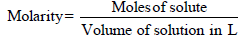(ii) N1V1 = N2V2

A 13% solution (by weight) contains 13g of solute (i.e. H2SO4) per 100 gm of solution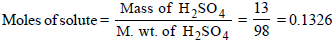Volume of solution in L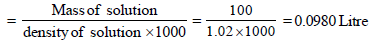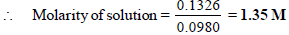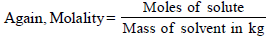Mass of solute in 100 ml of solution = 13 g [ 13 % solution]
Mass of solvent = Mass of solution – Mass of solvent
= 100 – 13 = 87 g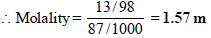N1 = 2.70, V1 = 100 ml, N2 = 1.5, V2 = ?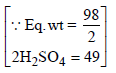N1V1 = N2V2; 2.70 × 100 = 1.5 × V2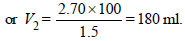∴ 100 ml of this acid should be diluted to 180 ml to prepare 1.5 N solution

2. A bottle of commercial sulphuric acid (density 1.787 g/ml) is labelled as 86 percent by weight. What is the molarity of the acid. What volume of the acid has to be used to make 1 litre of 0.2 M H2SO4?       (1979)

Ans : 15.81M; 12.65

Solution :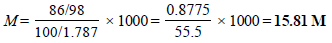M1V1 = M2V2
M1 = 15.81, V1 = ?
M2 = 0.2, V2 = 1 L = 1000 ml
∴ 15.81 × V1 = 0.2 × 1000∴ Amount of acid to be used to make 1 L of 0.2 M H2SO4 = 12.65.

3. 0.5 gm of fuming H2SO4 (Oleum) is diluted with water. This solution is completely neutralized by 26.7 ml of 0.4 N NaOH. Find the percentage of free SO3 in the sample of oleum.         (1980)

Ans : 3.84%

Solution :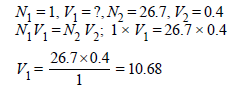49g (Q eq wt of H2SO4 = 49) of H2SO4 will be neutralised by = 1N 1000 ml NaOH
∴ 0.5g of H2SO4 will be neutralised by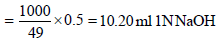Volume of 1 N NaOH used by dissolved
SO3 = 10.68 – 10.20 = 0.48 ml
SO3 + 2NaOH →   Na2SO4 + H2O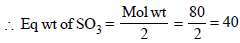Wt of SO3 in 0.48 ml of 1 M solution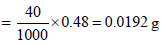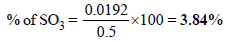4. The vapour pressure of pure benzene is 639.7 mm of mercury and the vapour of a solution of a solute in benzene at the same temperature is 631.9 mm of mercury. Calculate the molality of the solution. (1981 - 3 Marks)

Ans : 0.156 m/kg

Solution :

TIPS/Formulae :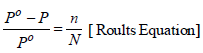Now the solution contains ‘m’ moles of solute per 1000 gm of benzene
Vapour pressure of benzene, Po = 639.7 mm
Vapour pressure of solution, P = 631.9 mm
Moles of benzene (Mol. wt. 78), N = 1000/ 78
Moles of solute, n = ?
Substitute these values in the Raoult’s equation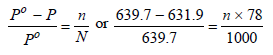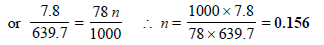Hence, molality of solution = 0.156 m

5. An organic compound CxH2yOy was burnt with twice the amount of oxygen needed for complete combustion to CO2 and H2O. The hot gases when cooled to 0ºC and 1 atm. pressure, measured 2.24 liters. The water collected during cooling weighed 0.9 g. The vapour pressure of pure water at 20ºC is 17.5 mm Hg and is lowered by 0.104 mm when 50 g of the organic compound are dissolved in 1000 g of water. Give the molecular formula of the organic compound. (1983 - 5 Marks)

Ans : (CH2O)5 or C5H10O5

Solution :  The chemical equation for the combustion of organic compound CxH2yOy can be represented as :
CxH2yOy + 2xO2 = x CO2 + y H2O + x O2
The gases obtained after cooling = x + x = 2x
∴ 2x = 2.24 litres                               [∵ H2O is in liquid state]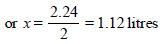Number of moles of CO2 =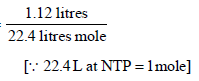=1/20 mole = 0.05 mole

The empirical formula of the organic compound is C(H2O) ...(1)
The mole fraction of the solute (A)
= relative decrease in vapour pressure of the solvent (B)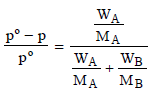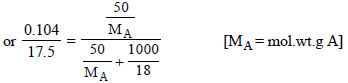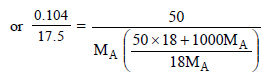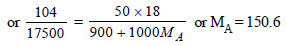Molecular wt. of the organic compound
(CH2O)n = 150
Molecular wt. of CH2O = 12 + 2 + 16 = 30

∴ 30 × n = 150                  [∵(CH2O)n = mol. formula]
or n = 150/30 = 5

∴ Molecular formula of the given organic compound is
(CH2O)5 or C5H10O5.

6. 'Two volatile and miscible liquids can be separated by fractional distillation into pure component', is true under what conditions? (1984 - 1 Mark)

Solution : If they form an ideal solution which obeys’ Raoult’s Law and for which
ΔHmixing = 0 and ΔVmixing = 0
Thus we can separate two volatile and miscible liquids by fractional distillation if, they should not form azeotropic solutions.

Offer running on EduRev: Apply code STAYHOME200 to get INR 200 off on our premium plan EduRev Infinity!

69 docs|36 tests

,

,

,

,

,

,

,

,

,

,

,

,

,

,

,

,

,

,

,

,

,

,

,

,

,

,

,

,

,

,

;